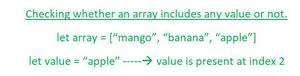Open in App
Not now

# How to check whether an array includes a particular value or not in JavaScript ?

• Difficulty Level : Basic
• Last Updated : 12 Jan, 2022

In this article, we will discuss the construction of an array followed by checking whether any particular value which is required by the user is included (or present) in the array or not.

But let us first see how we could create an array in JavaScript using the following syntax-

Syntax: The following syntax would be helpful for any user for the creation of an array with ease-

`let array = [item_1 , item_2, item_3 , ...];`

Now we have seen the creation of an array let us see several approaches to check whether an array includes any value in itself which a user wishes to have a look over it or not.Following are certain approaches that we may implement to check whether an array includes a value or not-

Approach 1: This is initial, traditional as the most common approach which any individual could think of in beginning. In this approach, we will run a for loop but before running the for loop, we will initialize our array and the value which we are looking for. Inside that for loop, we will see if our value is present in our array then along with the value name, we will return the index of that variable. If the value is not present in our array then we will exit from the for loop and then will print a message that the value is not present in the array.

Example:

## HTML

 `<``script``>``    ``let fruits_array = [``      ``"mango",``      ``"banana",``      ``"apple",``      ``"pineapple",``      ``"pomegranate",``      ``"orange",``    ``];``     ` `    ``let valueChecker = (value) => {``      ``for (let i = 0; i < ``fruits_array.length``; i++) {``        ``let ``current_value` `= ``fruits_array``[i];``        ``if (value === current_value) {``          ``return value + " is present at index: " + i;``        ``}``      ``}``      ``return value + " is not included in this array..";``    ``};``     ` `    ``console.log(valueChecker("apple"));``    ``console.log(valueChecker("app"));``    ``console.log(valueChecker("banana"));```

Output:

```apple is present at index: 2
app is not included in this array..
banana is present at index: 1```

Approach 2: After analyzing the above traditional most commonly used approach, now this is the newest approach. In this approach, we will be using .includes() method to check the value present in the array or not. If the value is present then we will print the message illustrating that value is present in an array. If the value is not present then we will print the message illustrating that value is not present.

Example:

## HTML

 `<``script``>``    ``let fruits_array = [``      ``"mango",``      ``"banana",``      ``"apple",``      ``"pineapple",``      ``"pomegranate",``      ``"orange",``    ``];``     ` `    ``let value_1 = "apple";``    ``let value_2 = "app";``    ``console.log(fruits_array.includes(value_1));``    ``console.log(fruits_array.includes(value_2));```

Output:

```true
false```

Approach 3: In this approach, we will be using indexOf() method. By using this method, we will be checking if the index value of the particular element which we are looking for, is greater than or equal to zero then we will print the message illustrating that the element is present at a certain index value. If our element is not present in the array, then we will be displaying an error message illustrating that the element is missing from the array.

## HTML

 `<``script``>``    ``let fruits_array = [``      ``"mango",``      ``"banana",``      ``"apple",``      ``"pineapple",``      ``"pomegranate",``      ``"orange",``    ``];``     ` `    ``let checkValue = (value) => {``      ``if (fruits_array.indexOf(value) >= 0)``        ``return value + " is present at index : "``          ``+ fruits_array.indexOf(value);``      ``else ``        ``return value + " is not present in this array";``    ``};``     ` `    ``console.log(checkValue("apple"));``    ``console.log(checkValue("app"));``    ``console.log(checkValue("mango"));```

Output:

```apple is present at index : 2
app is not present in this array
mango is present at index : 0```

My Personal Notes arrow_drop_up